Bombieri-Weil bound

Gauss sums (cf. Gauss sum) were first introduced by J.L. Lagrange in his work on algebraic equations. They were subsequently named for and developed by C.F. Gauss in the "Disquisitiones" as part of his work on the division of the circle. Since then, the study of special exponential sums has been synonymous with the study of cyclotomy. In the 19th century other exponential sums were studied in connection with the arithmetic of cyclotomic fields (cf. Cyclotomic field), most notably Jacobi sums, which are intimately connected with the multiplicative structure of finite fields (cf. also Finite field). Exponential sums capture the relation between additive and multiplicative properties of finite fields and, together with the language of harmonic analysis, provide a very powerful tool in many applications, particularly communication science and coding theory.

Other types of sums that have arisen in a natural way from the study of additive problems are the so-called Kloosterman sums, which appear in the study of partitions of integers and in the more general Hardy–Littlewood–Ramanujan methods. From the point of view of harmonic analysis, Kloosterman sums can be viewed as Fourier transforms of Gauss sums.

In the 20th century, the most significant breakthrough in the study of exponential sums came from the application of algebraic-geometric methods. First, H. Hasse discovered a close connection between bounds for certain cubic exponential sums that generalize quadratic Gauss sums and the number of rational points on certain elliptic curves (cf. also Elliptic curve). The formulation of this relation given by E. Artin establishes a correspondence between bounds for exponential sums and the so-called Riemann hypothesis (cf. Riemann hypotheses) for function fields in the hyper-elliptic case. The subsequent developments in algebraic geometry, leading to Weil's proof of the Riemann hypothesis for algebraic curves over finite fields, resulted in renewed interest in the study of exponential sums.

A. Weil was the first to realize that many sums, including Gauss and Kloosterman sums, could be viewed as constructions over the projective line. For example, ifis a rational function on the projective line, i.e., the quotient of two polynomials, then the sumwhere the poles ofhave been excluded, has a representation as a trace, namely as a sum of the Frobenius characteristic roots: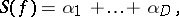whereis a subset of all the roots of the zeta-function of the Artin–Schreier covering (cf. also Artin–Schreier code; Artin–Schreier theory)This includes the special case whereis a polynomial of degree(and where the characteristic of the finite field does not divide) and the integercan then be calculated exactly as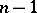. Using the bound(Weil's Riemann hypothesis), one obtains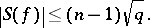Exponential sums of Weil–Bombieri type.

The methods of Weil were later studied by E. Bombieri, who not only saw how to replace the projective line by more general algebraic curves, but began the systematic study of exponential sums in several variables using Artin–Schreier coverings (see [a1] and Artin–Schreier theory). Bombieri's estimates were strengthened and applied to the solution of several significant problems in coding theory (see [a2]).

In a typical application of the Riemann hypothesis, one starts with an expression such as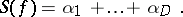It happens in many cases, especially in those that arise in the application of codes over the binary field, that the complex numbers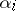not only satisfy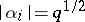, but also appear in complex-conjugate pairs. Furthermore, as algebraic integers, they are stable under the action of the Galois group of the rational numbers. This realization, first observed by J.-P. Serre, permits a finer utilization of the inequality of arithmetic-geometric means to obtain better Archimedean bounds for exponential sums than those found by Weil and Bombieri. A typical improvement has the following form: Ifis a polynomial of odd degree, in which case the genus of the Artin–Schreier covering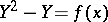is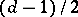, then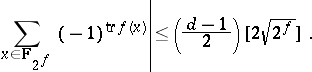This is a remarkable bound. It has application to coding theory (see [a5]) and improvements to it were suggested by coding theory. The exponential sum over the binary field has integer values and very often it also has divisibility properties that permit further improvements. In pursuing these ideas further, one is lead to consider, in addition to the Archimedean bounds,-adic bounds that reflect the divisibility properties that the sums satisfy.

To carry these ideas further, another great theme in the study of exponential sums has been incorporated, namely the Chevalley–Warning theorem. The methodology can best be described as follows.

By using improved versions of the Chevalley–Warning theorem and the Ax theorem (see [a3]), sharp bounds for the divisibility of the values of exponential sums over different field extensions are obtained. In turn, these are transferred, by arguments using formulas of Newton type, to divisibility properties of the roots of the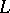-function associated with the exponential sum. Armed with this new information, one can pull off some further powers from the roots of the zeta-function without destroying their integral nature. Subsequently, Serre's old procedure can be applied to derive bounds.

These methods have many applications. One example is as follows. Letbe a polynomial of degree. Then (see [a6] for examples)A-adic Serre bound.

Looking at the original 1949 formulation of the Weil conjectures, one sees from the structure of the zeta-function of a non-singular algebraic variety as a kind of Euler–Poincaré characteristic that an algebraic variety of dimension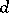has an internal skeleton very close to projective-dimensional space. More precisely, the difference between the number of rational points onover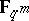and on the projective spaceis given by a formula of the type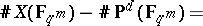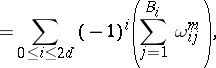where, foreven,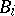is the dimension of the primitive part of theth cohomology group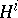and, forodd,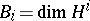. The boundis, of course, the Riemann hypothesis for non-singular projective algebraic varieties.

One of the principal aims of [a5] and [a6] is to describe a development of Serre's ideas called a-adic Serre bound. A surprising consequence of this development is that some of the inner sums in the above formula are empty, in the sense that the modified Betti numbers are zero (cf. Betti number). More precisely (see [a4], [a3] and [a6]), letbe a smooth projective variety (cf. Projective scheme) defined by the vanishing ofpolynomials. Letbe the integer defined by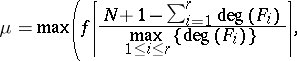Then, forodd, each eigenvalueof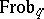acting on the étale cohomology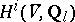is divisible by. Consequently,whenever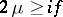. Furthermore, for the trace one has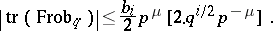(Here,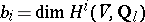; for the.) Foreven, each eigenvalueofacting on the primitive cohomologyis divisible by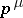. Consequently,whenever.

Further developments of the ideas described above work very well in situations that are geometrically very similar to curves, e.g., complete intersections.